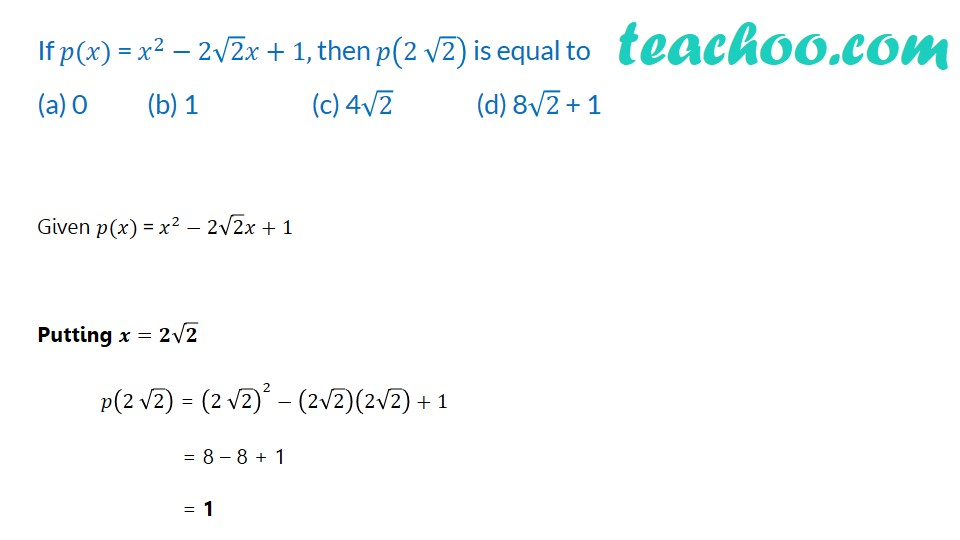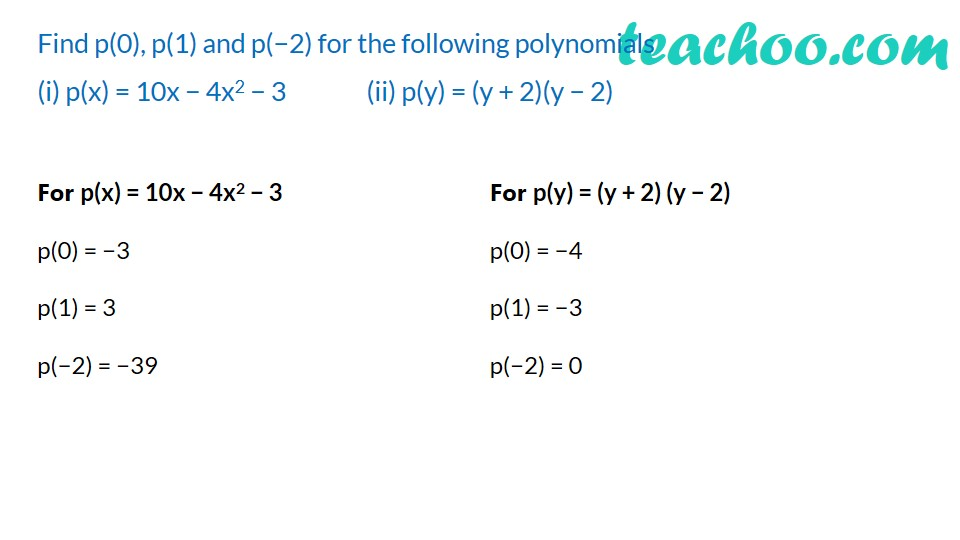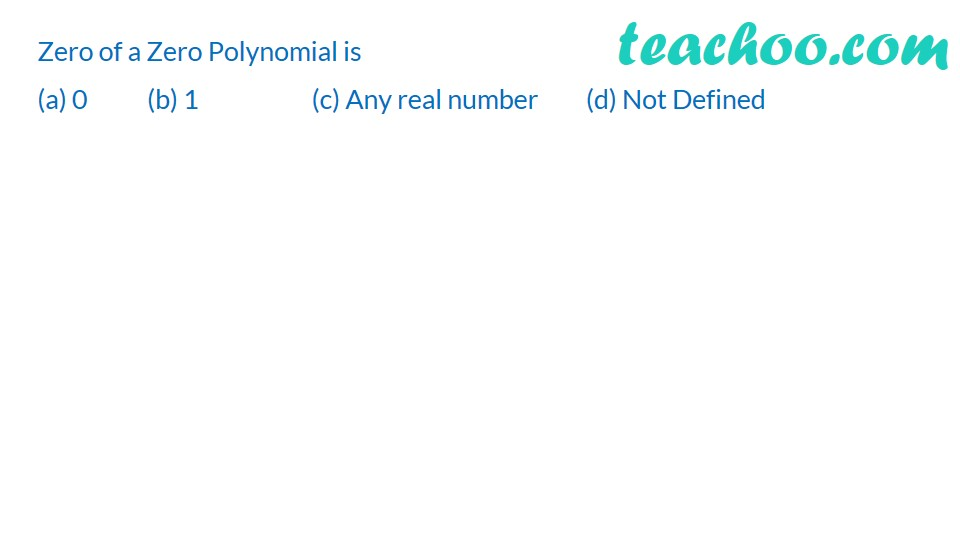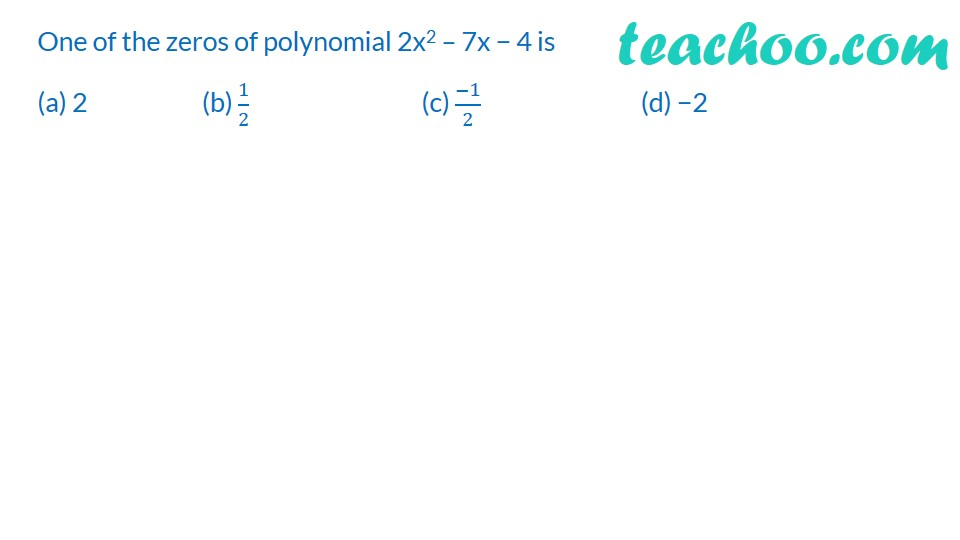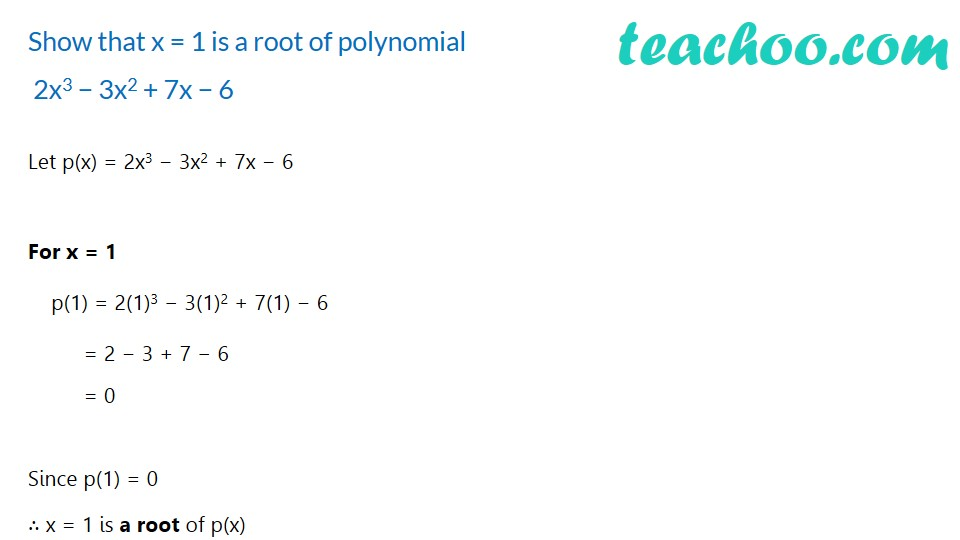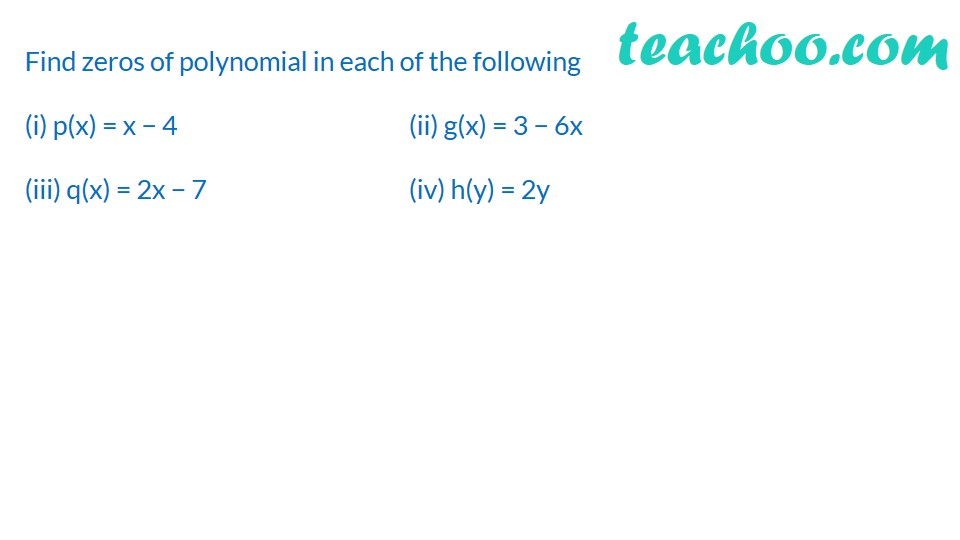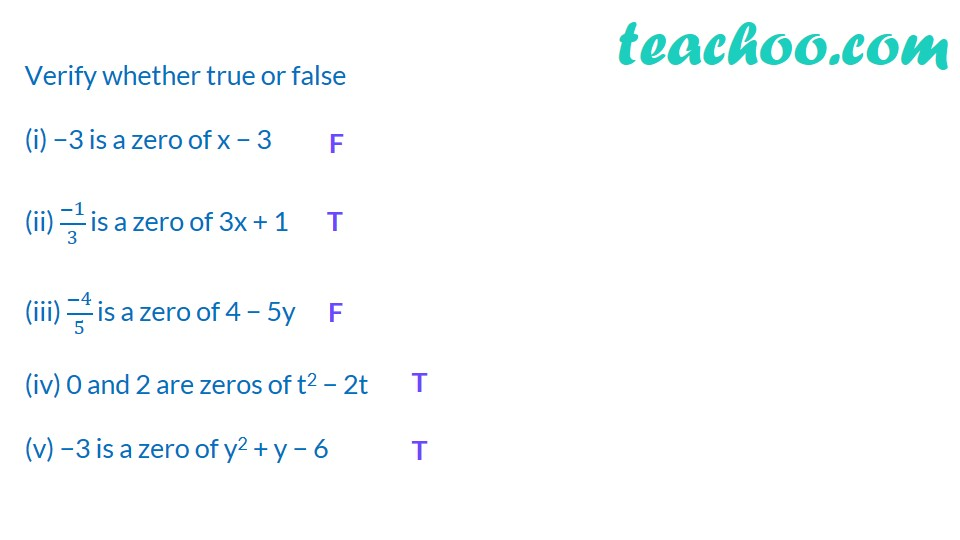1. Chapter 2 Class 9 Polynomials (Term 2)
2. Concept wise
3. Finding Zeroes of a polynomial

Transcript

If 𝑝(𝑥) = 𝑥^2−2√2 𝑥+1, then 𝑝(2 √2) is equal to (a) 0 (b) 1 (c) 4√2 (d) 8√2 + 1Given 𝑝(𝑥)" = " 𝑥^2−2√2 𝑥+1 Putting 𝒙=𝟐√𝟐 𝑝(2 √2) = (2 √2)^2−(2√2)(2√2)+1 = 8 – 8 + 1 = 1 Find p(0), p(1) and p(−2) for the following polynomials (i) p(x) = 10x − 4x2 − 3 (ii) p(y) = (y + 2)(y − 2)For p(x) = 10x − 4x2 − 3 p(0) = −3 p(1) = 3 p(−2) = −39 For p(y) = (y + 2) (y − 2) p(0) = −4 p(1) = −3 p(−2) = 0 Zero of a Zero Polynomial is (a) 0 (b) 1 (c) Any real number (d) Not DefinedOne of the zeros of polynomial 2x2 – 7x − 4 is (a) 2 (b) 1/2 (c) (−1)/2 (d) −2Show that x = 1 is a root of polynomial 2x3 − 3x2 + 7x − 6 Let p(x) = 2x3 − 3x2 + 7x − 6 For x = 1 p(1) = 2(1)3 − 3(1)2 + 7(1) − 6 = 2 − 3 + 7 − 6 = 0 Since p(1) = 0 ∴ x = 1 is a root of p(x) Find zeros of polynomial in each of the following (i) p(x) = x − 4 (ii) g(x) = 3 − 6x (iii) q(x) = 2x − 7 (iv) h(y) = 2yVerify whether true or false (i) −3 is a zero of x − 3 (ii) (−1)/3 is a zero of 3x + 1 (iii) (−4)/5 is a zero of 4 − 5y (iv) 0 and 2 are zeros of t2 − 2t (v) −3 is a zero of y2 + y − 6

Finding Zeroes of a polynomial